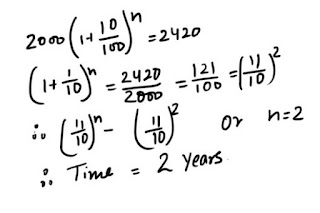# Compound Interest : 9 Questions that are repeated in the Exams

Compound Interest is an important chapter for banking exams. In every exams, you will find at least 1 question in every exam. From last 4 years I am analyzing the papers of various exams. I noticed that few questions are repeating in all the exams.### Ques 1.

What is the time in which Rs 2000 will amount to Rs. 2420 at 10% per annum compound interest?

### Ques 2.

What sum will become Rs. 6,690 after three years and Rs. 10,035 after six years on compound interest ?

### Ques 3.

The difference between the compound interest and the simple interest on a certain sum at 5% per annum for 2 years is Rs. 1.50. Find the sum.

### Ques 4.

Compound interest on a certain sum for 2 years at 10% p.a . is Rs 420. What would be the simple interest at the same rate and for the same time.

### Ques 5.

A sum of money placed at compound interest doubles itself in 4 years . In how many years will it amount to eight times itself ?

### Ques 6.

Find the least number of complete years in which a sum of money put at 25% p.a. compound interest will be more than double itself .

### Ques 7.

Divide Rs. 3903 between A and B , so That when compound annually A's share at the end of 7 years may be equal to B's share at the end of 9 years . The rate of interest is given to be 4% per annum.

### Ques 8.

How much more would Rs. 20,000 fetch , after two years , if it is put at 20 % p.a. . Compound interest payable half yearly than if it is put at 20% p.a. compound interest payable yearly ?
(a) Rs. 482
(b) Rs. 424
(c) Rs. 842
(d) Rs. 512

### Ques 9.

A sum in invested at compound interest payable annually . The interest in two successive years is Rs. 225 and Rs. 236.25. Th rate of interest is
(a) 4%
(b) 5%
(c) 3%
(d) 8%

### solutions :

Ans 1.Ans 2.

Now dividing the second equation by the first equation , we get :Substituting this value of (1+ R/100 )3 in the first equation we get : 3p/2 = 6,690
∴ Required sum = Rs. 4,460.

Ans 3.Ans 4.Ans 5.Hence , the sum will be 8 times itself in 12 years .
[ Note : The sum is 2 times in 4 years ; it will be 8 ( i.e. 23 ) times in 4 x 3 years i.e. 12 years ]

Ans 6.=> the least possible value of n is 4.
Hence, the least number of years required is 4.

Ans 7.Ans 8.Ans 9. 5%

#### Free QT Notes